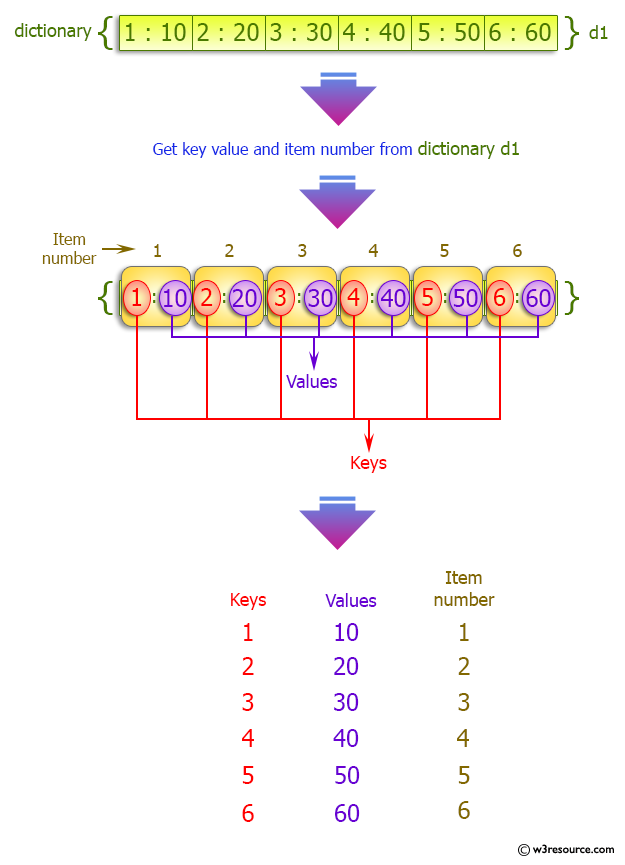﻿ Python: Get the key, value and item in a dictionary - w3resource# Python: Get the key, value and item in a dictionary

## Python dictionary: Exercise-31 with Solution

Write a Python program to get the key, value and item in a dictionary.

Sample Solution:-

Python Code:

``````dict_num = {1: 10, 2: 20, 3: 30, 4: 40, 5: 50, 6: 60}
print("key  value  count")
for count, (key, value) in enumerate(dict_num.items(), 1):
print(key,'   ',value,'    ', count)
```
```

Sample Output:

```key  value  count
1     10      1
2     20      2
3     30      3
4     40      4
5     50      5
6     60      6
```

Pictorial Presentation:## Visualize Python code execution:

The following tool visualize what the computer is doing step-by-step as it executes the said program:

Python Code Editor:

Have another way to solve this solution? Contribute your code (and comments) through Disqus.

What is the difficulty level of this exercise?

Test your Programming skills with w3resource's quiz.

﻿

## Python: Tips of the Day

Decapitalizes the first letter of a string:

Example:

```def tips_decapitalize(s, upper_rest=False):
return s[:1].lower() + (s[1:].upper() if upper_rest else s[1:])
print(tips_decapitalize('PythonTips'))
print(tips_decapitalize('PythonTips', True))
```

Output:

```pythonTips
pYTHONTIPS
```